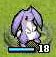# 星之一角

What have you found for these years?

## 2012-04-04

### thread-safe is not fiber-safe; fiber-safe could be even harder

Other posts:
2148. 04-04 thread-safe is not fiber-safe; fiber-safe could be even harder
2149. 04-04 thread-safe is not fiber-safe; fiber-safe could be even harder (2)
2151. 04-05 thread-safe is not fiber-safe; fiber-safe could be even harder (3)

## deterministic race conditions in fibers

``````i = 
a = Fiber.new{ t = i; Fiber.yield; i = t + 1 }
b = Fiber.new{ t = i; Fiber.yield; i = t + 1 }
p i # => 
a.resume
b.resume
a.resume # 1
b.resume # 1
p i # =>  # should be ``````

``````m = Mutex.new
i = 
a = Fiber.new{ m.synchronize{ t = i; Fiber.yield; i = t + 1 } }
b = Fiber.new{ m.synchronize{ t = i; Fiber.yield; i = t + 1 } }
p i # => 
a.resume

## reentrant mutex doesn't really lock for fibers. it still has race conditions

``````require 'monitor'
m = Monitor.new
i = 
a = Fiber.new{ m.synchronize{ t = i; Fiber.yield; i = t + 1 } }
b = Fiber.new{ m.synchronize{ t = i; Fiber.yield; i = t + 1 } }
p i # => 
a.resume
b.resume
a.resume # 1
b.resume # 1
p i # =>  # should be ``````

## detect deadlock and retry with threads to resume fibers again, finally fiber-safe now

``````require 'fiber'

def try_resume f
case r = f.resume
else
r
end if f.alive?
end

m = Mutex.new
i = 
a = Fiber.new{
begin
m.synchronize{ t = i; Fiber.yield; i = t + 1 }
Fiber.yield e
retry
end
}
b = Fiber.new{
begin
m.synchronize{ t = i; Fiber.yield; i = t + 1 }
Fiber.yield e
retry
end
}
p i # => 
while a.alive? || b.alive?
try_resume(a)
try_resume(b)
end
p i # => ``````

## retry with eventmachine instead, but do we really want to do this?

``````require 'fiber'
require 'eventmachine'

def try_resume f
case r = f.resume
else
r
end if f.alive?
end

EM.run{
m = Mutex.new
i = 
a = Fiber.new{
begin
m.synchronize{ t = i; Fiber.yield; i = t + 1 }
Fiber.yield e
retry
end
}
b = Fiber.new{
begin
m.synchronize{ t = i; Fiber.yield; i = t + 1 }
Fiber.yield e
retry
end
}
p i # => 
try_resume(a)
try_resume(b)
try_resume(a)
try_resume(b)
if !a.alive? && !b.alive?
p i # => 
EM.stop
end
}
}``````

## can STM (software transactional memory) help here?

STM for Ruby WANTED!!

## or let's just use threads, shall we?

``````m = Mutex.new
i = 
a = Thread.new{ m.synchronize{ t = i; sleep(0.1); i = t + 1 } }
b = Thread.new{ m.synchronize{ t = i; sleep(0.1); i = t + 1 } }
p i # => 
a.join
b.join
p i # => ``````
--
concurrency with shared state is really hard!

#### 0 retries: